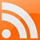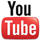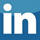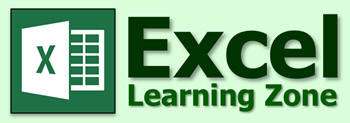Excel 2010-2019 Excel 2007 Excel 2003 Tips & Tricks Excel Forum Course Index CIG Excel Book

 Home   Courses   Templates   Seminars   TechHelp   Forums   Help   Contact   Join   Order   Logon More... What's New? Popular Links ------------ Access Courses Access Index Access TechHelp Access Forum ------------ Excel Courses Excel Index ------------ Code Vault Testimonials

 < Previous: Excel Expert 4 Next: Excel Expert 6 >

Excel Expert Level 5
Microsoft Excel Expert Tutorial - 1 Hour, 5 Minutes

This is the fifth class in our Excel Expert Series. It picks up where Expert Level 4 left off. The focus of this course is on math and basic statistical functions. Topics covered include: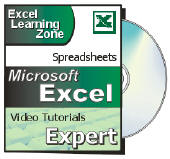- More with the SUM function  - Calculating a Running Balance / Total  - The SUMIF and SUMIFS functions  - Counting Numeric, Blank, Non-Blank Cells  - Rounding Up, Down, and more  - Lots of Math (Median, Sqrt, Modulus, Random)  - Automatic Recalculation  - Basic Trigonometry in Excel  - Calculate the Height of a BuildingOrder Now

If you would like a preview of what's covered in this class,
Excel Expert Level 5
 Description: Excel Expert Level 5 Versions: Recorded with Microsoft Excel 2010. Valid for 2007 to 2021. Pre-Requisites: Running Time: 1 Hour, 5 Minutes Cost: \$9.99

This course is for the expert user who has good experience with Microsoft Excel or has completed the five courses in our Beginner Series plus Expert Level 1, 2, 3, and 4 classes. This course will teach you how to work with math and basic statistical functions in Excel.

We will begin by learning more about the SUM function. You'll see how to use multiple, non-contiguous ranges in your functions. You will learn how to create a running balance - also called a running total.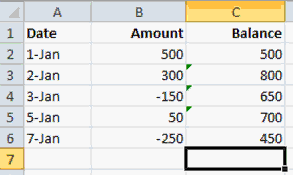Next you'll learn how to use the SUMIF function to add up values IF they meet a certain criteria. For example, below you will see the total sales for each sales rep. The rep's name is the condition. You will learn how to use static and variable criteria. You will learn the difference between thesum range and criteria range. We will also discuss the related functions COUNTIF and AVERAGEIF.In the next lesson, we'll learn about the SUMIFS function (and the related COUNTIFS and AVERAGEIFS functions). SUMIFS is similar to SUMIF, but it allows you to use multiple criteria (instead of just one). In the example below, you'll see that we have a list of orders, their due dates, and whether or not they're paid. We can use SUMIFS to add up all of the orders that are both past due and unpaid.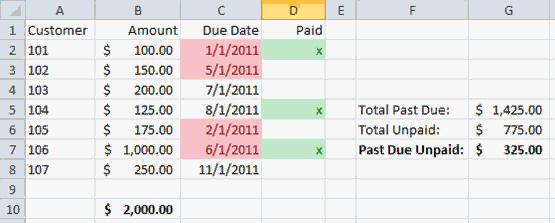Next we'll learn about the various counting and rounding functions. You will see how to COUNT numeric cells, blank cells, and non-blank cells. You will learn all of the many functions that you can use to ROUND different numbers, and how these functions treat fractions and negative numbers differently.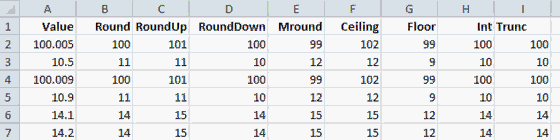Next you will learn a ton of different mathematical functions including MEDIAN, MODE, PRODUCT, POWER, SQRT, QUOTIENT, MOD, ABS, SIGN, and more. You'll learn about scientific notation, integer division, calculating a remainer, and how to generate RANDOM numbers. You'll also learn how to use (and disable) automatic recalculation.Finally, we'll look at some of the basic trigonometry functions in Excel. You'll learn about SIN, COS, TAN, PI, DEGREES, and RADIANS. As an example, we'll learn how to calculate the height of a building knowing it's distance and the angle to the top.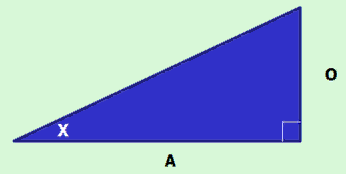Again, this is the perfect class for anyone who wants to learn how to work effectively with math and basic statistics functions in Microsoft Excel . Of course, if you have any questions about whether or not this class is for you, please contact me.

Complete Outline - Excel Expert Level 5

 00. Intro (5:49) 01. More With SUM (5:01) Non-contiguous ranges with SUM Insert function with multiple ranges Running balance Running total 02. SUMIF (11:05) Add up values with specific criteria ">=100" Variable criteria ">="&A1 SUMIF, COUNTIF, AVERAGEIF Sum Range vs. Criteria Range Make sure to use absolute references 03. SUMIFS (10:03) Sum of commissions by sales rep Commissions by rep over \$100 Orders past due Unpaid orders past due SUMIFS, COUNTIFS, AVERAGEIFS 04. Counting & Rounding (11:29) COUNT, COUNTA, COUNTBLANK What you see isn't necessarily valid Decrease decimal hides true value ROUND, ROUNDUP, ROUNDDOWN MROUND, CEILING, FLOOR INT, TRUNC 05. Misc Math (13:03) MEDIAN MODE PRODUCT Scientific Notation 4.19E+23 POWER SQRT 10^2 Integer Division QUOTIENT MOD Modulus ABS SIGN Random Numbers RAND RANDBETWEEN Turn off Automatic Recalculation F9 to Recalculate 06. Trigonometry (5:10) SIN, COS, TAN PI RADIANS DEGREES Calculate Height of a Building 07. Review (3:08)

Keywords: microsoft excel tutorial, microsoft excel tutorial, microsoft office excel tutorial, microsoft excel training, COUNT, COUNTA, COUNTBLANK, ROUND, ROUNDUP, ROUNDDOWN, MROUND, CEILING, FLOOR, INT, TRUNC, MEDIAN, MODE, PRODUCT, Scientific Notation, POWER, SQRT, QUOTIENT, Integer division, MOD, Modulus, ABS, SIGN, RAND, RANDBETWEEN, Trigonometry, SIN, COS, TAN, PI, DEGREES, RADIANS

 Learn  Access - index Excel - index Word - index Windows - index PowerPoint - index Photoshop - index Visual Basic - index ASP - index Seminars More... Customers  Login My Account My Courses Lost Password Memberships Student Databases Change Email Info  Latest News New Releases User Forums Topic Glossary Tips & Tricks Search The Site Code Vault Collapse Menus Help  Customer Support Web Site Tour FAQs TechHelp Consulting Services About  Background Testimonials Jobs Affiliate Program Richard Rost Free Lessons Mailing List Order  Video Tutorials Handbooks Memberships Learning Connection Idiot's Guide to Excel Volume Discounts Payment Info Shipping Terms of Sale Contact  Contact Info Support Policy Mailing Address Phone Number Fax Number Course Survey Email Richard [email protected]